# Orifice Plates

## Orifice Plates General Description and Quick Guide

Orifice Element in Flow Simulator models the pressure loss across orifice plates using Loss characteristic curves obtained from Internal Flow Systems by DS Miller. Orifice Plates have a total of 7 sub-categories like Sharp Edge, Sharp Edge Conical, Orifice Long, Orifice Short, Orifice Radiused, Orifice Square and Screens. The mass flow rate calculations remain same for most of the cases. All the orifice plates elements can be used in Compressible (e.g. gas systems) and Incompressible (e.g. hydraulic and non-hydraulic systems)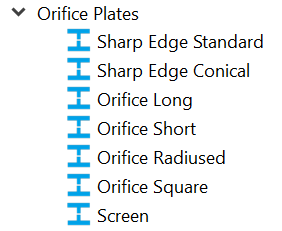Orifice diameter (area) and Pipe diameter (area) are mandatory inputs. Users can choose forward and reverse contraction coefficient from the drop down (By Default Miller curves are chosen). User can provide their own fixed or curve values for Contraction coefficient.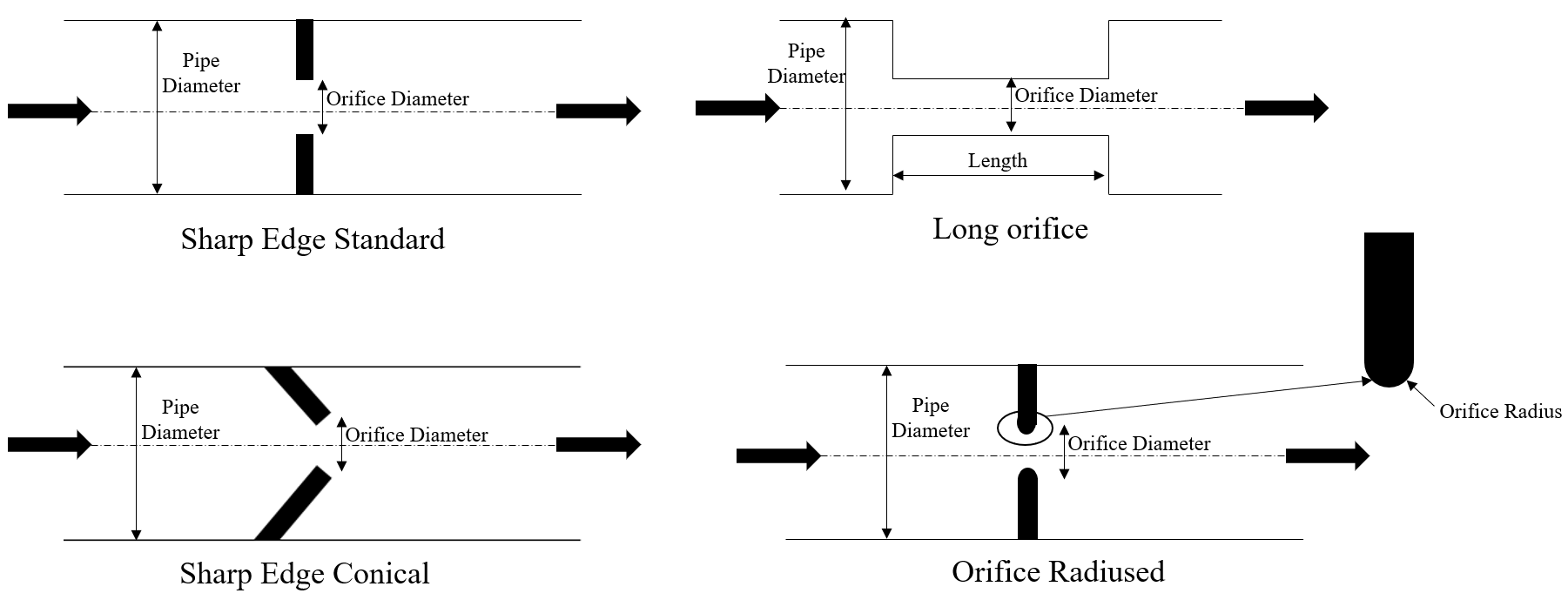## Orifice Plates Element Theory

K loss values for various Orifice Plate Configurations

 Subtype Curve Reference Orifice: Sharp-Edged Forward Contraction Coefficient 90o (Miller, 2nd Ed., Fig 14.2) Reverse Contraction Coefficient 90o (Miller, 2nd Ed., Fig 14.2) Orifice: Sharp-Edged Conical Forward Contraction Coefficient 45o (Miller, 2nd Ed., Fig 14.2) Reverse Contraction Coefficient 45o (Miller, 2nd Ed., Fig 14.2) Orifice: Long Loss Coefficient for Thin Edged Sharp Orifice & Square Holes (Miller, 2nd Ed., Fig 14.3) Correction Factor for Edged Radii (Miller, 2nd Ed., Fig 14.4) Correction Factor for orifice length (Miller, 1st Ed., Fig 14.5) Orifice: Short Loss Coefficient for Thin Edged Sharp Orifice & Square Holes (Miller, 2nd Ed., Fig 14.3) Orifice: Radiused Loss Coefficient for Thin Edged Sharp Orifice & Square Holes (Miller, 2nd Ed., Fig 14.3) Correction Factor for Edged Radii (Miller, 2nd Ed., Fig 14.4) Orifice: Square Loss Coefficient for Thin Edged Sharp Orifice & Square Holes (Miller, 2nd Ed., Fig 14.3) Screens Loss Coefficient for Screens (Miller, 2nd Ed., Fig 14.7)

Apart from K Loss reference from “Internal Flow Systems” by DS Miller, Flow Simulator provides additional K Loss option from “Applied Fluid Dynamics, RD Blevins” (For Orifice Short Subtype) & I. E. Idelʹchik, Handbook of Hydraulic Resistance (For Orifice Long Subtype)

K Loss Calculation from IdelCheck for Long Orifice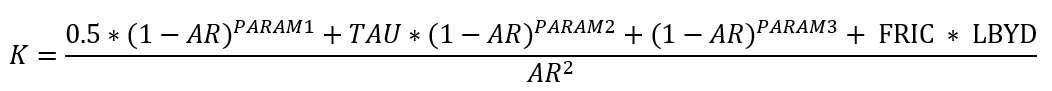Where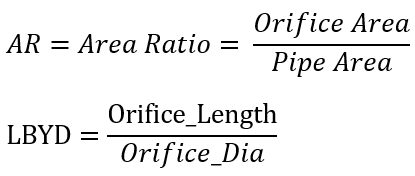FRIC=Friction factor (Refer Solver General theory for Friction calculation)

PARAM1 = 1.0

PARAM2 = 1.5

PARAM3 = 2.0

IF (LBYD <= 0.2D0) THEN

TAU = 1.35D0 - 0.65D0 * LBYD

ELSEIF (LBYD <= 0.4D0) THEN

TAU = 1.34D0 - 0.60D0 * LBYD

ELSEIF (LBYD <= 0.6D0) THEN

TAU = 1.62D0 - 1.30D0 * LBYD

ELSEIF (LBYD <= 0.8D0) THEN

TAU = 2.10D0 - 2.10D0 * LBYD

ELSEIF (LBYD <= 1.0D0) THEN

TAU = 1.14D0 - 0.90D0 * LBYD

ELSEIF (LBYD <= 1.4D0) THEN

TAU = 0.59D0 - 0.35D0 * LBYD

ELSEIF (LBYD <= 2.0D0) THEN

TAU = 0.286666667D0 - 0.133333333D0 * LBYD

ELSEIF (LBYD <= 3.0D0) THEN

TAU = 0.06D0 - 0.02D0 * LBYD

ELSE

TAU = 0.0D0

ENDIF

K Loss Calculation from RD Blevins for Short Orifice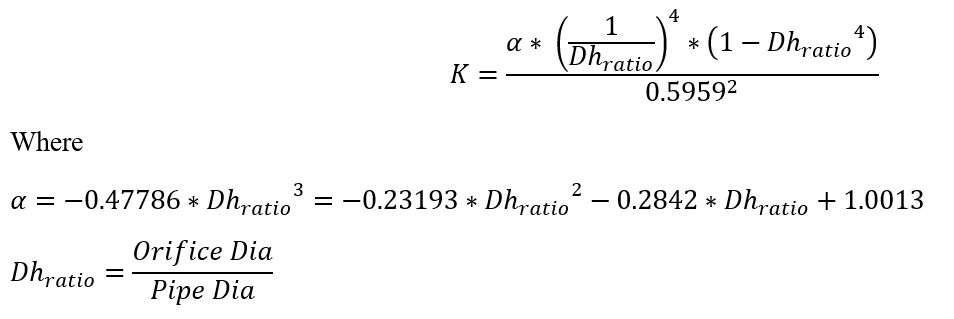Mass Flow rate Calculation:

 Nomenclature: W: Mass flow rate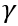: Specific heat Ratio A: Orifice mechanical area R: Gas Constant CD: Coefficient of Discharge Ts: Static Temperature K: Incompressible Loss Coefficient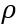: Density Tt: Total Temperature MN: Vena Contracta Mach Number Pt: Total pressure Cp: Specific Heat Ps: Static pressure gc: Gravitational Constant Subscripts: in: Inlet station Corr: Correction ex: exit station

Incompressible Liquid Calculation:

The Incompressible total loss coefficient is given by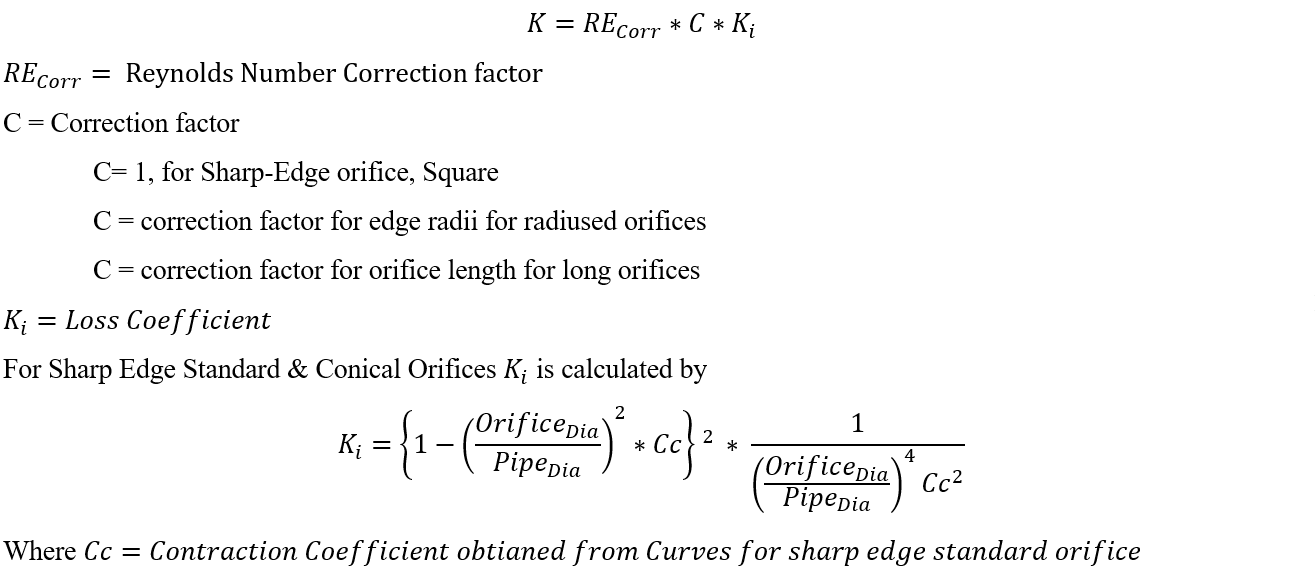For all other subtypes, it is obtained directly from curve referenced above.

Mass flow rate across the element is calculated as follows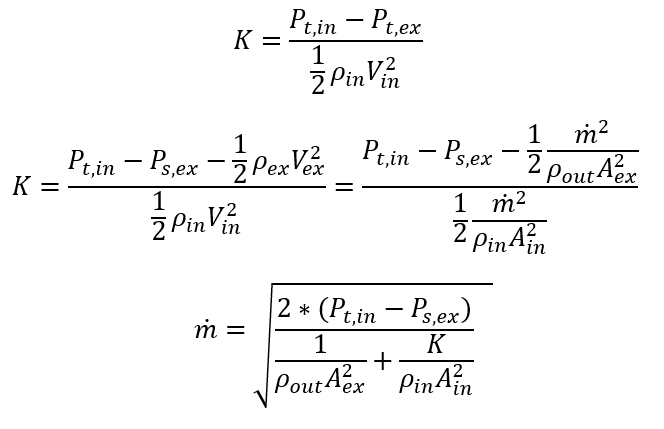Compressible Gas Calculation:

Compressible Loss Coefficient, K is derived from incompressible loss coefficient, Ki with Compressibility correction factor. To obtain Compressibility correction coefficient, it is required to calculate the Mach number at Vena Contracta.

An iterative procedure is used to calculate mass flow rate & Mach Number at Vena contracta. The Compressibility Correction Coefficient is estimated using Curve (Fig 7.14 2 Ed., D.S. Miller)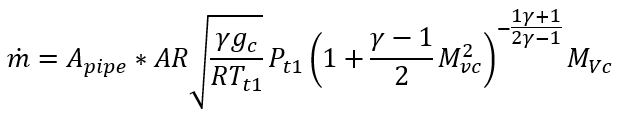For Additional Momentum loss, Reynolds Number correction, Portion of Upstream Dynamic Head loss, Exit K Loss refer Solver General theory section.

Note: Reynolds Number is calculated based on orifice diameter & orifice Velocity

## Orifice Plates Outputs

The following listing provides details about conventional orifice output variables. A graphical depiction of a conventional orifice output entry is provided.

Name Description Units
Pipe Area Usually an echo of the user input unless modified inside Flow Simulator. in2, m2
Orifice Area Usually an echo of the user input unless modified inside Flow Simulator. in2, m2
Pipe Diameter Usually an echo of the user input unless modified inside Flow Simulator. in, m
Pipe Diameter Usually an echo of the user input unless modified inside Flow Simulator. in, m
DQ_IN

Portion of Ustrm Chamb. Dyn. Head Lost

(Usually an echo of the user input unless modified inside Flow Simulator.)

flag
ELEMENT_THETA

Tangential Angle

(Usually an echo of the user input unless modified inside Flow Simulator.)

unitless
ELEMENT_PHI

(Usually an echo of the user input unless modified inside Flow Simulator.)

unitless
REL_INLET_ANGLE (Usually an echo of the user input unless modified inside Flow Simulator.) deg

(Usually an echo of the user input unless modified inside Flow Simulator.)

in, m
Orif_Length

Orifice length

(Usually an echo of the user input unless modified inside Flow Simulator.)

in, m
K_from Table_or_Eqn Loss Value (Can be a User Input or Calculated by Flow Simulator)
K_BeforeFilletCorrection Uncorrected Loss Value (Calculated)
FilletCorrection Calculated Fillet Correction
K_AfterFilletCorrection Corrected Loss Value (User Input or Calculated)
K_Loss_Input Input Loss Value (Calculated) – If Constant Value is Provided
K_Loss_Incompr Loss Value (Calculated)
K_Loss_Compr Loss Value (Calculated)
ELEMENT_RPM

RPM (Rotor index)

(Usually an echo of the user input unless modified inside Flow Simulator.)

(Usually an echo of the user input unless modified inside Flow Simulator.)

in, m
Element Theta Exit Exit Tangential Angle rad
PTS Driving pressure relative to the rotational reference frame (i.e. rotor) at the restriction inlet. psi, mPa
PSIN Static Pressure inlet. psi, mPa
PTEX Total pressure relative to the rotational reference frame (i.e. rotor) at the restriction exit including supersonic effects. psi, mPa
PSEX

Static pressure relative to the rotational reference frame (i.e. rotor) at the restriction exit.

Limited by critical pressure ratio for supersonic flows.

psi, mPa
PSEB Effective sink (static) pressure downstream of the restriction. psi, mPa
TTS Total temperature of fluid relative to the rotational reference frame (i.e. rotor) at the restriction inlet. deg F, K
TSIN Static Temperature Inlet deg F, K
TTEX Total Temperature Exit deg F, K
TSEX Static Temperature Exit deg F, K
QIN Fraction of total heat to be added before the restriction. (0 = None, 1 = All)

BTU/s, W
INVEL Inlet Velocity ft/s, m/s
INMN Inlet Mach Number
INREYN Inlet Reynolds Number
EXVEL Exit Velocity ft/s, m/s
EXMN Exit Mach Number
EXREYN Exit Reynolds Number
PTVC Total Pressure at Vena Contracta psi, mPa
PSVC Static Pressure at Vena Contracta psi, mPa
TTVC Total Temperature at Vena Contracta deg F, K
TSVC Static temperature at Vena Contracta deg F, K
VCVEL Velocity at Vena Contracta ft/s, m/s
VCMN Mach Number at Vena Contracta ft/s, m/s
VEX Fluid velocity relative to the rotational reference frame (i.e. rotor) at the restriction exit including heat input (QIN) effects. ft/s, m/s
VABS Magnitude of the fluid total absolute velocity ft/s, m/s
VTAN_ABS Magnitude of the fluid absolute tangential velocity ft/s, m/s
VAXIAL Magnitude of the fluid axial velocity ft/s, m/s
THTA_ABS Fluid absolute tangential flow angle rad
VREL Magnitude of the fluid total velocity relative to the element ft/s, m/s
VTAN_REF Reference frame tangential velocity ft/s, m/s
VTAN_REL Magnitude of the fluid tangential velocity relative to the element ft/s, m/s
VNORM Magnitude of the fluid total velocity relative to the element ft/s, m/s
THTA_REL Fluid relative tangential flow angle rad
TTABS Absolute total temperature deg F, K
TTREL Relative total temperature deg F, K

## References

1. Blevins, R. D., Applied Fluid Dynamics Handbook, Krieger Publications, 2003
2. Miller, D, Internal Flow Systems, Miller Innovations, 1990
3. I. E. Idelʹchik, Handbook of Hydraulic Resistance, CRC-Press, 1994